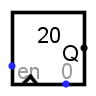#Zufallsgenerator

 Bibliothek: Memory Eingeführt: 2.3.0 Aussehen:## Verhalten

This component iterates through a pseudorandom sequence of numbers, which steps forward to the following number in the sequence each time the clock is triggered while the component is enabled. Technically speaking, the algorithm used to compute the pseudorandom sequence is a linear congruential generator: Starting from a seed r0, the following number r1 is the number

r1 = (25,214,903,917 r0 + 11) mod 248

The next value r2 is computed from r1 using the same computation, and so forth. This sequence is of 48-bit numbers; the value seen from the component is the low-order bits as configured by its Data Bits attribute, after first throwing out the lower 12 bits of the current seed.

Besides the clock input, the component also includes an enable input, which leads the clock input to be ignored when enable is 0, and the reset input, which resets the component's value asynchronously to the initial seed r0.

The initial seed is user-configurable. If it is configured at 0 (which is the default), then the seed is based on the current time; when instructed to reset through the reset input, the component starts with the same seed based on the past time. It acquires a new seed only when the entire simulation is reset.

## Pins

East edge, labeled Q (output, bit width matches Data Bits attribute)
Outputs the value currently stored by the component.
West edge, top pin, labeled with a triangle (input, bit width 1)
Clock: At the instant that this is triggered as specified by the Trigger attribute, the component steps to the following number in its sequence.
West edge, bottom pin (input, bit width 1)
Enable: The component is enabled when this input is disconnected or 1; but if it is 0, then the clock input is ignored.
Südseite (Eingang, Bitbreite 1)
Reset: When this is 1, the pseudorandom sequence asynchronously resets to its initial seed.

## Attribute

When the component is selected or being added, Alt-0 through Alt-9 alter its Data Bits attribute.

Data Bits
The bit width of the value emitted by the component.
Seed
The starting value used for the pseudorandom sequence. If this is 0 (the default), then the starting value is based on the time that the current simulation began.
Trigger
Configures how the clock input is interpreted. The value rising edge indicates that the component should update its value at the instant when the clock rises from 0 to 1. The falling edge value indicates that it should update at the instant the clock falls from 1 to 0.
Label
The text within the label associated with the component.
Label Font
The font with which to render the label.

Keines.

## Verhalten des Textwerkzeugs

Allows the label associated with the component to be edited.

Zurück zur Bibliotheksreferenz